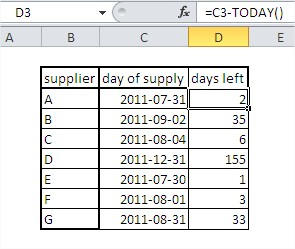#### How to Use TODAY Function in Excel

In this lesson, you will learn how to use the TODAY function. This function is really easy and is more powerful than you thought.

The function, which returns the current system date, is mainly used in various forms. As well as in logistics, e.g., to calculate how many days were left until delivery or transportation day.

## What is the TODAY function in Excel?

TODAY stands for “Today’s Date”. It is a function that takes no arguments and returns the current date in the form of a serial number. The serial number for today’s date is always increasing, so you can use the TODAY function to track the passage of time.

## How does the TODAY function work?

The TODAY function works by retrieving the current date from the computer’s operating system. The date is then converted into a serial number and returned by the function.

## Today function syntax

Syntax is just =TODAY()

Excel will display the current date, e.g., 2013-07-30

As you see above, the TODAY function has no arguments. Its correct result depends on correctly setting the date (and time) in Windows.## How do I calculate how many years have passed?

• Just use this formula: =YEAR(TODAY())-1986

The answer is 27 years. Yes, I am 27 years old.

• =INT((TODAY()-A1)/365.25) – write your date to the A1 cell. It could date from the future as well.
• YEAR(TODAY())-YEAR(A1) – another way to calculate the year difference

## How do I calculate the time difference using the TODAY function?

• =DATEVALUE(“2/11/2020”)-TODAY() – difference between your date and the current date Remember about the proper formatting of cells? It must be GENERAL or NUMBER.
• =A1<TODAY()-10 – checks if A1 date is earlier than 10 days ago. It could be useful for checking on some payments from clients. Displays TRUE or FALSE.
• =DATEDIF(A1,TODAY(),”y”) & ” Years, ” & DATEDIF(A1,TODAY(),”ym”) & ” Months” – displays how many years and months passed from A1 date to current date.
• =DATEDIF(A1,TODAY(),”y”) & ” Years, ” & DATEDIF(A1,TODAY(),”ym”) & ” Months, ” & DATEDIF(A1,TODAY(),”md”) & ” Days” – gives you years, months, and days between two dates.

## Today function examples

• =DAY(TODAY()) – extracts current day
• =MONTH(TODAY()) – extracts current month
• =WEEKDAY(TODAY()) – extracts the number of the day of the week
• =TEXT(WEEKDAY(TODAY()),”dddd”) – displays name of the day of the week
• =DATE(YEAR(TODAY()),MONTH(TODAY())+1,1)-1 – displays the last day of current month
• =IF(TODAY()=A1,TRUE,FALSE) – checks if A1 is current date and displays TRUE or FALSE

Note that the TODAY function is a volatile function, which means it will recalculate every time the worksheet is opened or recalculated, even if there are no changes to the worksheet.